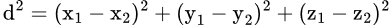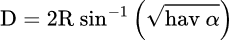# Using Haversines with SQL To Calculate Accurate Distances

Sometimes it becomes desirable to know the distance between two geographical places. This could come about as a desire to know where a customer base is regarding a store, where the nearest fire station is to a house, or wanting to know how many people would be affected by a location closing or opening.

There are a lot of services out there that will take your data and geocode it for distances between a single location and a data set. But if you need to have a lot of distances calculated, or you aren't sure of where your one of your points are, this can become onerous and costly.

Programming your own solution can be difficult, though. Using standard three-dimensional math doesn't take into account the curve of the earth. Looking into how to calculate the distance between two points on a sphere will get you a lot of equations, but these are often intricate and difficult to debug.

This article will show you how to calculate the distances between points in a database, taking into account the curvature of the earth.

### Why You Can't Use 3D Geometry

There's no built in way in a database to calculate the distance between two places. Most people tackle this by using the regular geometry distance formula:Approaching the problem in this way, though, is like tunneling under the earth's crust. The further apart the points, the less accurate this calculation. This standard distance formula doesn't take into account the shape of the earth, which is mostly spherish.

### Points on a Sphere

In order to calculate a better distance, it is necessary to use analytic geometry to calculate the distance between two points on the surface of a sphere. This wouldn't be bad if there was somewhere to quickly grab a formula. Unfortunately, most found formulas are intricate, long and very difficult to translate into a programming language.

This is where we can rely on a mathematical function called a haversine. It's an application of a more general trigonometric law for relating points on a spherical surface.

The haversine formula, generalized, is:### Distance Using Haversines

The standard distance between two latitude/longitude pairs can be expressed aswhere:
R is the radius of the earth (pick your favorite one), andand your data points are in latitude/longitude pairs ofEasy peasy, right?

In order to make this calculation work, you will have to have latitude and longitude for your data points. Latitude and longitude should be in decimal degrees, and I'm sure you remember from trigonometry that we will need to convert values to radians to make trig work right. We'll take care of the conversion to radians inside the function.

Here are the table structures:

``CREATE TABLE dbo.SiteOne ( SiteOnePK INT IDENTITY PRIMARY KEY , SiteName VARCHAR(50) , LatitudeDegree NUMERIC(30, 15) , LongitudeDegree NUMERIC(30, 15) ) CREATE TABLE dbo.SiteTwo ( SiteOnePK INT IDENTITY PRIMARY KEY , SiteName VARCHAR(50) , LatitudeDegree NUMERIC(30, 15) , LongitudeDegree NUMERIC(30, 15) );``

### The Data

Here is the sample data:

``INSERT INTO dbo.SiteOne (SiteName,LatitudeDegree,LongitudeDegree) VALUES ('Great Pyramid at Giza',29.97925,31.134222) , ('Statue of Liberty',40.68925,-74.044444) , ('Eiffel Tower',48.858361,2.294444) , ('Uluru',-25.343667,131.036889); INSERT INTO dbo.SiteTwo (SiteName,LatitudeDegree,LongitudeDegree) VALUES ('Mt. Kilimanjaro',-3.067389,37.355583) , ('Taj Mahal',27.175056,78.042111) , ('Golden Gate Bridge',37.820111,-122.478278) , ('Machu Picchu',-13.162889,-72.545028) , ('Brandenburg Gate',52.51625,13.377667);``

As you can see from the sample data, we will be finding the distance between some of the world's notable places.

### The Function

The function, using the haversines, is fairly straightforward:

``CREATE FUNCTION dbo.ufnSphericDistance ( @LatitudeOne NUMERIC(30, 15) , @LongitudeOne NUMERIC(30, 15) , @LatitudeTwo NUMERIC(30, 15) , @LongitudeTwo NUMERIC(30, 15) ) RETURNS NUMERIC(30, 15) AS /***************************************************************************************** * Created on 03/12/2022 * Created by LMoss * * Description: Calculate the distance between two latitude/longitude pairs IN DECIMAL DEGREES * *****************************************************************************************/ BEGIN DECLARE @Result NUMERIC(30, 15) --convert the input parameters into radians DECLARE @LatitudeOneInRadians NUMERIC(30, 15) = @LatitudeOne * PI() / 180 DECLARE @LongitudeOneInRadians NUMERIC(30, 15) = @LongitudeOne * PI() / 180 DECLARE @LatitudeTwoInRadians NUMERIC(30, 15) = @LatitudeTwo * PI() / 180 DECLARE @LongitudeTwoInRadians NUMERIC(30, 15) = @LongitudeTwo * PI() / 180 --use your favorite Earth radius here. If you want kilometers, you can use that as well - your output will be in km DECLARE @EarthRadiusInMiles NUMERIC(30, 15) = 3958.939 --calculate the haversine of the difference in latitudes DECLARE @HavTheta NUMERIC(30, 15) = (1 - COS(@LatitudeOneInRadians - @LatitudeTwoInRadians)) / 2 --calculate the haversine of the difference in longitudes DECLARE @HavPhi NUMERIC(30, 15) = (1 - COS(@LongitudeOneInRadians - @LongitudeTwoInRadians)) / 2 --calculate the haversine of the alpha DECLARE @HavAlpha NUMERIC(30, 15) = @HavTheta + COS(@LatitudeOneInRadians) * COS(@LatitudeTwoInRadians) * @HavPhi --do your final calculation SET @Result = 2 * @EarthRadiusInMiles * ASIN(SQRT(@HavAlpha)) RETURN @Result END GO``

### Getting the Results

Because we want to calculate the distance from all of the points in table 1 to all the points in table 2, we are going to use a CROSS JOIN.

``SELECT f.SiteName , g.SiteName , dbo.ufnSphericDistance(f.LatitudeDegree, f.LongitudeDegree, g.LatitudeDegree, g.LongitudeDegree) DistanceInMiles FROM dbo.SiteOne f CROSS JOIN dbo.SiteTwo g``

#### And the results:

 Site Name 1 Site Name 2 Distance in Miles Great Pyramid at Giza Mt. Kilimanjaro 2320.1288 Great Pyramid at Giza Taj Mahal 2833.2633 Great Pyramid at Giza Golden Gate Bridge 7452.1931 Great Pyramid at Giza Machu Picchu 7480.0686 Great Pyramid at Giza Brandenburg Gate 1798.9758 Statue of Liberty Mt. Kilimanjaro 7471.3509 Statue of Liberty Taj Mahal 7417.9789 Statue of Liberty Golden Gate Bridge 2566.1218 Statue of Liberty Machu Picchu 3722.2347 Statue of Liberty Brandenburg Gate 3969.3014 Eiffel Tower Mt. Kilimanjaro 4157.3643 Eiffel Tower Taj Mahal 4200.2581 Eiffel Tower Golden Gate Bridge 5560.9655 Eiffel Tower Machu Picchu 6234.3274 Eiffel Tower Brandenburg Gate 546.2652 Uluru Mt. Kilimanjaro 6357.4330 Uluru Taj Mahal 5060.3882 Uluru Golden Gate Bridge 8133.6025 Uluru Machu Picchu 9338.9041 Uluru Brandenburg Gate 8741.4261

### If You Want To Dive Into The Math

The background on the math is easy to find on the internet. I highly recommend that any programmer understand the math of any equation they are trying to put into use. It's really the only way to make sure that your results are accurate. A thorough and easy-to-follow explanation of math behind using haversines to calculate distance between latitude and longitude points can be found in Distance between Points on the Earth's Surface.